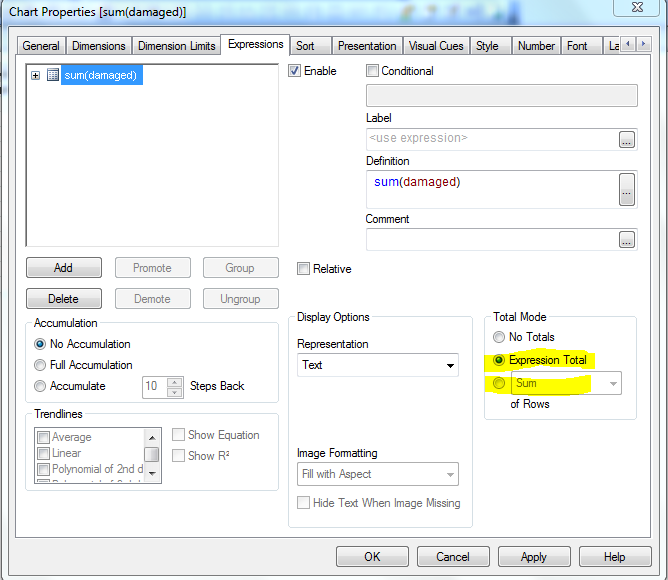# QlikView Layout & Visualizations

Discussion Board for collaboration on QlikView Layout & Visualizations.

Valued Contributor II

## Difference between sum and expression Total in Straight table

Hi,

Can anyone please tell me the difference between sum and Expression total in the expression tab of Straight table?1 Solution

Accepted SolutionsMVP & Luminary

## Re: Difference between sum and expression Total in Straight table

Hi,

Sum - will give you the sum of all rows in the table

Expression Total - will give you the overall expression total.

For example:

Year     A     B

2013     50     100

2014     50     150

When you use A/B as expression and use Year as Dimension

Sum Mode - 83% (50 + 33) (50/100 + 50/150 = 50% + 33 % = 83%)

Expression Mode - 40% (100/250) (50 + 50) / (100 + 150)

Regards,

Jagan.

11 RepliesNot applicable

## Re: Difference between sum and expression Total in Straight table

Grand total of the expression. u can suppress the Grand total by selecting No Total Option.

Valued Contributor II

## Re: Re: Difference between sum and expression Total in Straight table

Hi Raja,

Thanks for the reply.

I want to know the difference between 'expression total' and 'sum', as highlighted in the below picture. The Problem is not about showing the total or hiding it...Not applicable

## Re: Difference between sum and expression Total in Straight table

Hi Sundarakumar,

I lot cases the output of the both options is same. Except the some Situation Like, when you are having for one parent node as two child's (Value 100) the total of parent in Expression Total is 10 and sum total will be 20.MVP & Luminary

## Re: Difference between sum and expression Total in Straight table

Hi,

Sum - will give you the sum of all rows in the table

Expression Total - will give you the overall expression total.

Regards,

Jagan.

Valued Contributor II

## Re: Difference between sum and expression Total in Straight table

Do u mean it like, Expression total will return in the sum without considering what dimension we are using?Not applicable

## Re: Difference between sum and expression Total in Straight table

Hi Sundar,

PFA...

Reagrds,

raja

Valued Contributor II

## Re: Difference between sum and expression Total in Straight table

Thanks for the reply, sorry i dont understand what u r trying to explain.

Can u please elaborate?MVP & Luminary

## Re: Difference between sum and expression Total in Straight table

Hi,

Sum - will give you the sum of all rows in the table

Expression Total - will give you the overall expression total.

For example:

Year     A     B

2013     50     100

2014     50     150

When you use A/B as expression and use Year as Dimension

Sum Mode - 83% (50 + 33) (50/100 + 50/150 = 50% + 33 % = 83%)

Expression Mode - 40% (100/250) (50 + 50) / (100 + 150)

Regards,

Jagan.

Valued Contributor II

## Re: Difference between sum and expression Total in Straight table

Difference is shown significantly when using distinct in expressions,

Expression Total Calculates the Expression regardless of all dimensions just similar as you write your expression in a text box.

Sum of Rows > Sums the values calculated by expression in all rows of the chart.